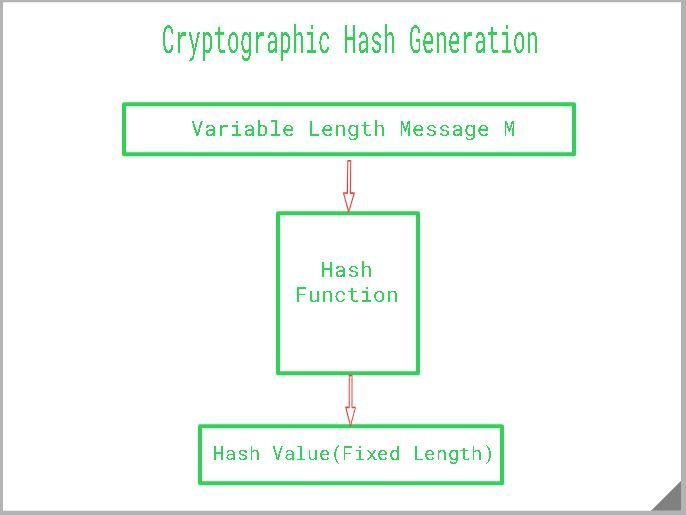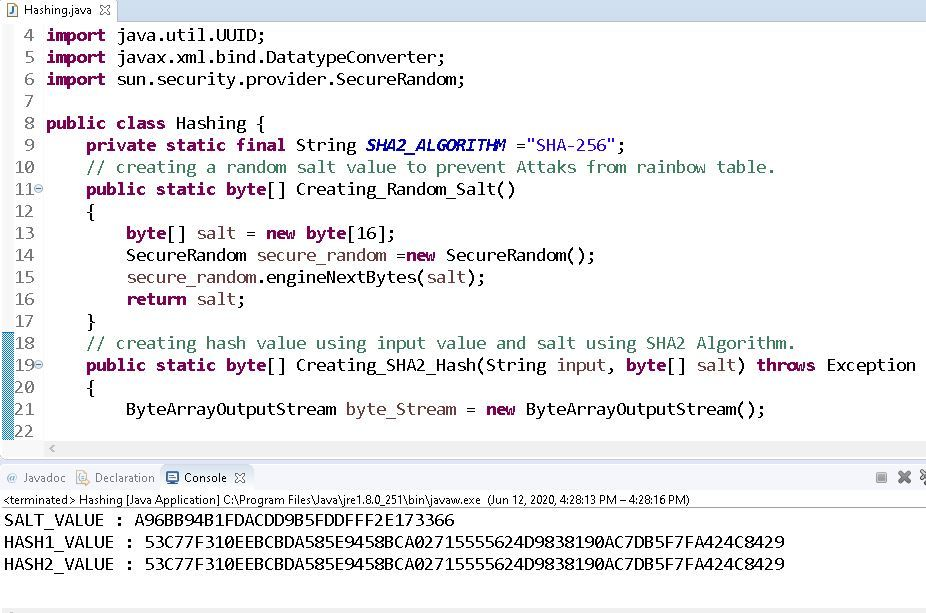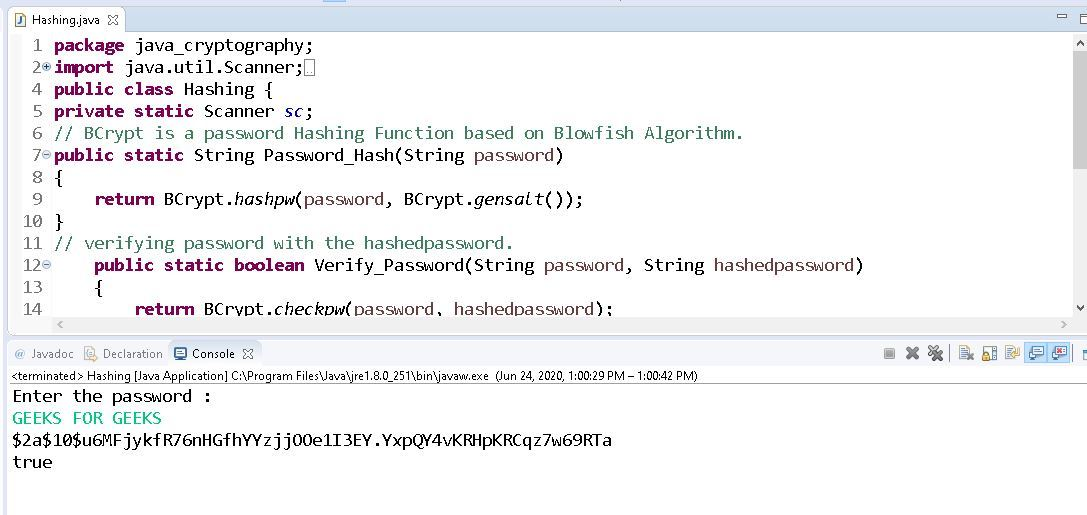GeeksforGeeks App
Open AppBrowser
Continue

# Cryptographic Hash Function in Java

Cryptographic Hash is a Hash function that takes random size input and yields a fixed-size output. It is easy to calculate but challenging to retrieve the original data. It is strong and difficult to duplicate the same hash with unique inputs and is a one-way function so revert is not possible. Hashing is also known by different names such as Digest, Message Digest, Checksum, etc.### Properties Of Cryptography Hash Function

The ideal cryptographic hash function has the following main properties:

1. Deterministic: This means that the same message always results in the same hash.
2. Quick: It is quick to compute the hash value for any given message.
3. Avalanche Effect: This means that every minor change in the message results in a major change in the hash value.
4. One-Way Function: You cannot reverse the cryptographic hash function to get to the data.
5. Collision Resistance: It is infeasible to find two different messages that produce the same hash value.
6. Pre-Image Resistance: The hash value shouldn’t be predictable from the given string and vice versa.
7. Second Pre-Image Resistance: Given an input, it should be difficult to find another input that has the same hash value.

We often hear the term Cracking a Hash, there are a couple of ways to do that:

• Find an algorithm to generate a collision between two hashes. The more advance the algorithm is, the more difficult it is to crack the hash.
• Another way is to find an algorithm to identify a unique and different input that will produce a given hash. It is similar to a collision, but instead of colliding, we are focusing on finding the input using an algorithm.
• Some common hashes we still use today that are considered “cracked” from a cryptographic point of view are MD5(Message-Digest Algorithm) and SHA-1(Secure Hash Algorithm 1). Keep in mind that these are technically broken Hashes and never use for security purposes.

### How to create a Cryptographic Hash

• Create a random salt value using SecureRandom class, SecureRandom class generates strong random values. The engineNextBytes(byte[] bytes) method is used to generate a user-specified number of random bytes.
• Convert two sets of bytes into one using ByteArrayOutputStream class and create it to ByteArray.
• Create an instance of a message-digest passing SHA2_ALGORITHM which returns a hash of the given input value.
• UUID is used to genmessage-digested to a string and passed as input.
• The returned object can be converted to a hex binary format using DatatypeConverter.

## Java

 `// Java program to demonstrate``// how to create a Hash` `package` `java_cryptography;` `import` `java.io.ByteArrayOutputStream;``import` `java.security.MessageDigest;``import` `java.util.UUID;``import` `javax.xml.bind.DatatypeConverter;``import` `sun.security.provider.SecureRandom;` `public` `class` `Hashing {` `    ``// Initializing the final string variable``    ``private` `static` `final` `String SHA2_ALGORITHM``        ``= ``"SHA-256"``;` `    ``// Creating a random salt value to prevent``    ``// attacks from the Rainbow table.``    ``public` `static` `byte``[] Creating_Random_Salt()``    ``{``        ``byte``[] salt = ``new` `byte``[``16``];``        ``SecureRandom secure_random``            ``= ``new` `SecureRandom();``        ``secure_random.engineNextBytes(salt);``        ``return` `salt;``    ``}` `    ``// Creating hash value using input value``    ``// and salt using the SHA2 Algorithm.``    ``public` `static` `byte``[] Creating_SHA2_Hash(``        ``String input, ``byte``[] salt) ``throws` `Exception``    ``{``        ``ByteArrayOutputStream byte_Stream``            ``= ``new` `ByteArrayOutputStream();` `        ``byte_Stream.write(salt);``        ``byte_Stream.write(input.getBytes());``        ``byte``[] valueToHash``            ``= byte_Stream.toByteArray();``        ``MessageDigest messageDigest``            ``= MessageDigest``                  ``.getInstance(SHA2_ALGORITHM);``        ``return` `messageDigest``            ``.digest(valueToHash);``    ``}` `    ``public` `static` `void` `main(String args[])``        ``throws` `Exception``    ``{` `        ``// Calling the function Creating_Random_Salt()``        ``// to generate a random salt value``        ``byte``[] salt = Creating_Random_Salt();``        ``System.out.println(``            ``"SALT_VALUE: "``            ``+ DatatypeConverter.printHexBinary(salt));``        ``String valueToHash``            ``= UUID.randomUUID().toString();` `        ``// Generating first hash with the salt``        ``byte``[] hash1``            ``= Creating_SHA2_Hash(valueToHash, salt);` `        ``// Generating second hash with exact salt``        ``// to check if we get the same hash.``        ``byte``[] hash2``            ``= Creating_SHA2_Hash(valueToHash, salt);` `        ``// Print first and the second hash value``        ``System.out.println(``            ``"HASH1_VALUE: "``            ``+ DatatypeConverter``                  ``.printHexBinary(hash1));``        ``System.out.println(``            ``"HASH2_VALUE: "``            ``+ DatatypeConverter``                  ``.printHexBinary(hash2));``    ``}``}`

Note: Salt is a random value added to the input data(passwords) to defend against pre-computed hash attacks such as Rainbow tables.

Output:

SALT_VALUE: A96BB94B1FDACDD9B5FDDFFF2E173366 HASH1_VALUE: 53C77F310EEBCBDA585E9458BCA02715555624D9838190AC7DB5F7FA424C8429 HASH2_VALUE: 53C77F310EEBCBDA585E9458BCA02715555624D9838190AC7DB5F7FA424C8429### How to create Cryptographic Hashing Passwords

As we have seen how to generate a Hash now, let us use Bcrypt to hash a password. Do not use broken Hashing algorithms for Hashing Passwords. Bcrypt is a password Hashing function based on Blowfish Cipher.

Approach:

• Pass the password to hashpw function which is in Bcrypt class which can also generate the salt by itself and returns a string.
• Verify if the password hash and password are really matching using the checkpw() function. It returns a Boolean value.

Code:

## Java

 `// Java program to demonstrate``// how to hash a password` `package` `java_cryptography;` `import` `java.util.Scanner;``import` `org.springframework``    ``.security``    ``.crypto``    ``.bcrypt``    ``.BCrypt;` `public` `class` `Hashing {` `    ``// Creating a private instance``    ``// of Scanner class``    ``private` `static` `Scanner sc;` `    ``// BCrypt is a password Hashing``    ``// Function based on Blowfish``    ``// Algorithm.``    ``public` `static` `String Password_Hash(``        ``String password)``    ``{``        ``return` `BCrypt.hashpw(``            ``password, BCrypt.gensalt());``    ``}` `    ``// Verifying password with the``    ``// hashed password.``    ``public` `static` `boolean` `Verify_Password(``        ``String password,``        ``String hashed_password)``    ``{``        ``return` `BCrypt.checkpw(``            ``password, hashed_password);``    ``}` `    ``public` `static` `void` `main(``        ``String args[]) ``throws` `Exception``    ``{` `        ``// Scanner class instance connected``        ``// to the Input Stream(System.in)``        ``sc = ``new` `Scanner(System.in);` `        ``System.out.println(``            ``"Enter the password: "``);` `        ``// Scanner class instance``        ``// reading the user input``        ``String p = sc.nextLine();` `        ``// Generate hashed password``        ``String passwordHash``            ``= Password_Hash(p);` `        ``// Print Hashed Password``        ``System.out.println(``            ``"Hashed-password: "``            ``+ passwordHash);` `        ``// Printing the result of verification``        ``// of hashed password``        ``// with original password``        ``System.out.println(``            ``"Verification: "``            ``+ Verify_Password(``                  ``p, passwordHash));``    ``}``}`

Output:

Input: Enter the password: GEEKS FOR GEEKS Output: Hashed-password: \$2a\$10\$u6MFjykfR76nHGfhYYzjjOOe1I3EY.YxpQY4vKRHpKRCqz7w69RTa Verification: true### Hash Uses

My Personal Notes arrow_drop_up